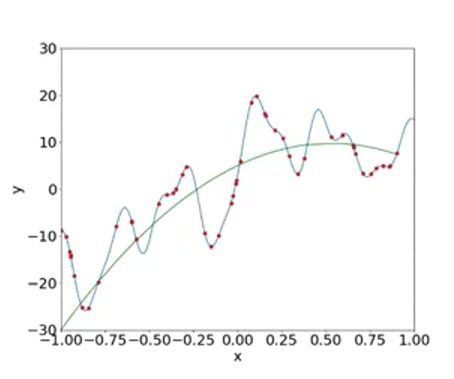Search

# How Math plays a key role in Machine Learning process

Updated: Jan 27, 2020

Many people including me had a doubt that how these machines are smart enough to figure out things on their own. The answer came out to be mathematics. It is just amazing how math folds up and show us these kinds of miracles in the name of machine learning.

Let's begin our journey to have an understanding of how machines learn!

Here we have an image and we have the output as one of the several classes that the image could belong to. In this case, I am considering 5 classes.Our data would look in the form of numbers, where numbers are the pixel values of the image. Suppose we have a 30x30 image i.e. we have 900 numerical values and we have 1 of the labels associated with the image.

Now, if we come to the equation y = f(x), we understand that there is some relation exist between the input and output. This image is classified as a dog because there is some function that takes this image and gives us the output y (which is a dog).

The problem is that in most cases we don't know what this function is. So take any application where you want to use M.L, it would typically be the case that we don't know what this function is.

If you already knew this function then there is nothing to learn.

What Machine typically does in M.L is that it looks at lots of this data, where we have an x & y and in all of these cases it realizes that there is some relation between x & y.

NOW, what we do is, we say that we don't know what the true relation is, but here are our approximations of what the relationship between y & x. So, we come up with some function which we believe is the best approximate relationship between x & y.Just see the data shown above. From this data, our job is to come up with a function that best explains the relationship between y & x. and just as in this case, in the real world also these functions could be very complex and not really expressible.

So, let's see how we proceed in this:

start with linear function -> y = mx + c (not fitting)Ploynomial degree 2 function -> y = ax^2 + bx + c (not fitting)No matter what value of a,b,c,d we try, we will not be able to get a situation where for any given x here, the output of the function(green curve) would be the same as the red dots.

We have to discover the function which can capture the relationship between x & y.

Here comes the magic: What Machines do :

The machine is trying to very efficiently find the best values of a,b,c and so on(by permutation) so that across all these points my error is as low as possible i.e. I should be as close to the predictions as possible.

y = ax^25 + bx^24 + .......cx + d is the function which is fitting the curve(found by machine)Now, this Explains and tries to model the relationship between x & y almost perfectly.

--

Thank you for the long read, I will be making these kinds of content regularly. Please consider subscribing and sharing it with all your friends.

344 views1 comment

See All